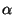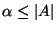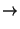Next: Simulation Results Up: Host Diversity Previous: Diversity and Core Sizes

## Modeling Diversity

To better understand the impact of diversity skew, we simulate a system of hosts with various attributes . On the Internet, most hosts run some version of Windows with Internet Explorer as the web browser , so we biased the attribute distribution towards having some fixed subset of attributes. The size of this subset depends on the valuechosen for the diversity of the system. To see this, consider a subset of size. Assuming that each attribute haspossible values, for such a subset the total number of distinct configurations is. Thus, there is some integer,, that satisfies the following equation:(1)

In our simulations, we compute the value ofusing Equation 1, and then pick a subsetof attributes such that. For every attributein, we fix the value offor a fractionof the hosts. We then randomly choose values for the remaining attributes for this fraction of hosts. For the remaining hosts, we pick attribute configurations at random, but we make sure that each configuration is not a configuration of any host in the first fraction.Next: Simulation Results Up: Host Diversity Previous: Diversity and Core Sizes
2003-06-20